Courses

# Circular Motion MCQ Level - 1

## 10 Questions MCQ Test Topic wise Tests for IIT JAM Physics | Circular Motion MCQ Level - 1

Description
This mock test of Circular Motion MCQ Level - 1 for Physics helps you for every Physics entrance exam. This contains 10 Multiple Choice Questions for Physics Circular Motion MCQ Level - 1 (mcq) to study with solutions a complete question bank. The solved questions answers in this Circular Motion MCQ Level - 1 quiz give you a good mix of easy questions and tough questions. Physics students definitely take this Circular Motion MCQ Level - 1 exercise for a better result in the exam. You can find other Circular Motion MCQ Level - 1 extra questions, long questions & short questions for Physics on EduRev as well by searching above.
QUESTION: 1

### A stone tied to a string is rotated in a vertical plane. If mass of the stone is m, the length of the string is R and the linear speed of the stone is v  when the stone is at its lowest point, then the tension in the string at the lowest point will be :

Solution: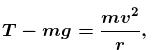(centripetal force at lowest point)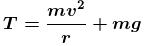The correct answer is: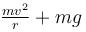QUESTION: 2

### A ring of radius R lies in vertical plane. A bead of mass m can move along the ring without friction. Initially the bead is at rest at the bottom most point on ring. The minimum constant horizontal speed v with which the ring must be pulled such that the bead completes the vertical circle :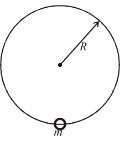Solution:

In the frame of ring (inertial w.r.t. earth), the initial velocity of the bead is v at the lowest position.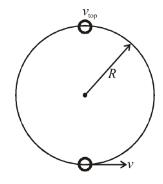The condition for bead to complete the vertical circle is, its speed at top position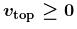From conservation of energy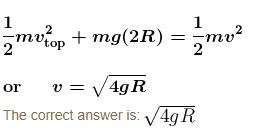QUESTION: 3

### When the road is dry and coefficient of friction is μ the maximum speed of a car in a circular path is 10ms–1. If the road becomes wet and coefficient of friction become μ/4, what is the maximum speed permitted?

Solution: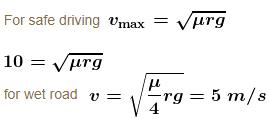QUESTION: 4

A car of mass M is traveling on a horizontal circular path of radius r. At an instant its speed is v and tangential acceleration is :

Solution: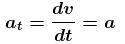friction force on car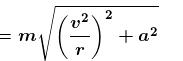which is greater than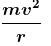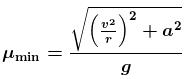∴   it is not less than a/g for safe turn.

The correct answer is: The magnitude of frictional force on the car is greater than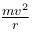QUESTION: 5

A body is tied up by a string of length l  and rotated in vertical circle at minimum speed. When it reaches at highest point string breaks and body moves on a parabolic path in presence of gravity according to figure. In the plane of point A, value of horizontal range AC will be :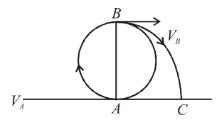Solution: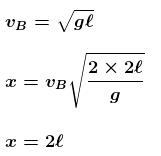The correct answer is: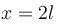QUESTION: 6

The maximum velocity at the lowest point, so that the string just slack at the highest point in a vertical circle of radius l.

Solution: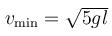The correct answer is: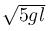QUESTION: 7

A section of fixed smooth circular track of radius R in vertical plane is shown in the figure. A block is released from position A and leaves the track at B. The radius of curvature of its trajectory when it just leaves the track at B is: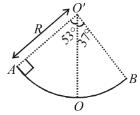Solution:

By energy conservation between A and B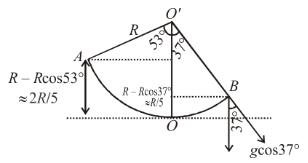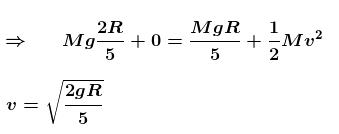Now, radius of curvature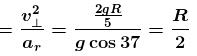QUESTION: 8

A particle of mass m begins to slide down on a fixed smooth sphere from the top. What is its tangential acceleration when it breaks off the sphere?

Solution:

At loose contact N = 0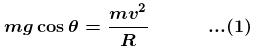from energy conservation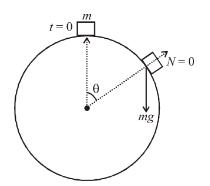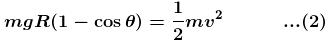from (1) & (2)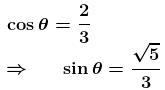tangential acceleration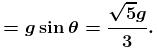The correct answer is: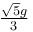QUESTION: 9

A particle is projected horizontally from the top of a tower with a velocity v0. If v  be its velocity at any instant, then from radius of curvature of the path of the particle at that instant is directly proportional to :

Solution: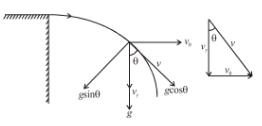As we know :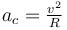(centripetal acceleration)

From figure :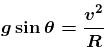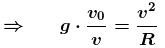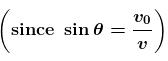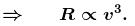QUESTION: 10

The breaking tension of a string is 10N. A particle of mass 0.1kg tied to it is rotated along a horizontal circle of radius 0.5metre. The maximum speed with which the particle can be rotated without breaking the string is :

Solution: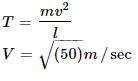Track your progress, build streaks, highlight & save important lessons and more!

### Similar Content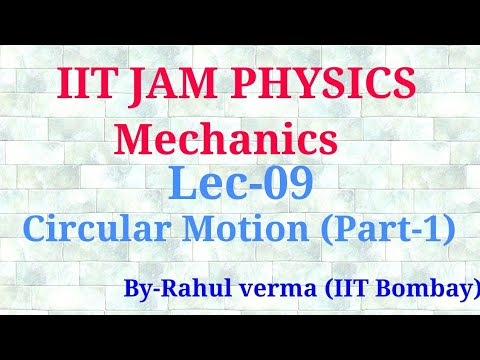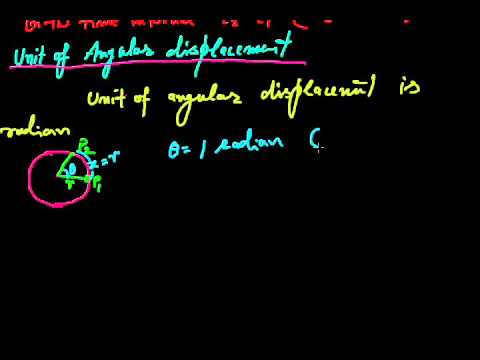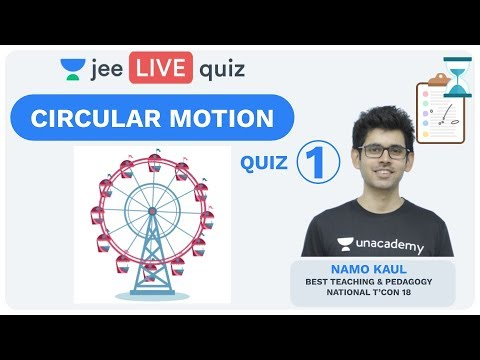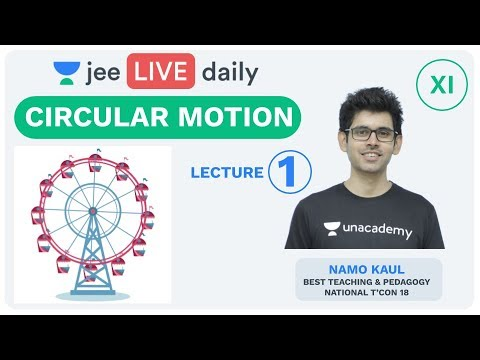### Related tests